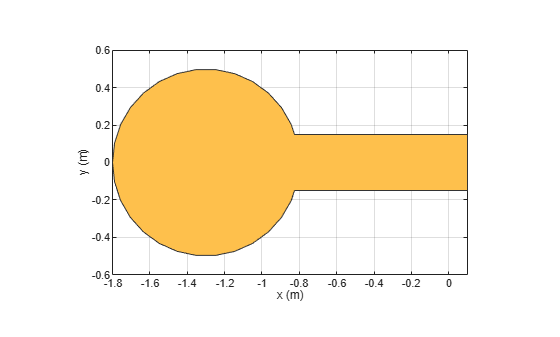# mirrorY

Mirror shape along y-axis

Since R2022a

## Syntax

``mirrorY(shape)``
``mirroredshape = mirrorY(shape)``

## Description

example

````mirrorY(shape)` mirrors a shape along the y-axis.```

example

````mirroredshape = mirrorY(shape)` stores the mirrored shape in a shape object.```

## Examples

collapse all

Create a Dumbell shaped patch by performing operations on a circular and a rectangular shape.

Create patch shape and view it

Use `antennna.Circle` and `antenna.Rectangle` to create a patch shape and view it.

```c1 = antenna.Circle(Center=[-1.3 0],Radius=0.5); c2 = antenna.Rectangle(Center=[-0.4 0],Length=1,Width=0.3); cm = c1 + c2; show(cm)```Mirror the shape along y-axis

Use `mirrorY` to create a mirror image of the shape along y-axis and view it.

```cmm = mirrorY(cm); show(cmm)```Add both shapes to create Dumbell shape

Add the original shape and its mirror image along y-axis to create a Dumbell shape and view it.

```c3 = c1 + c2 + cmm; show(c3)```## Input Arguments

collapse all

Shape to mirror, specified as `antenna.Shape` object. You can specify rectangular, circular, elliptical, triangular, or polygon shape.

Example: `antenna.Polygon`

Complex Number Support: Yes

## Output Arguments

collapse all

Mirrored shape stored as an `antenna.Shape` object.

## Version History

Introduced in R2022a